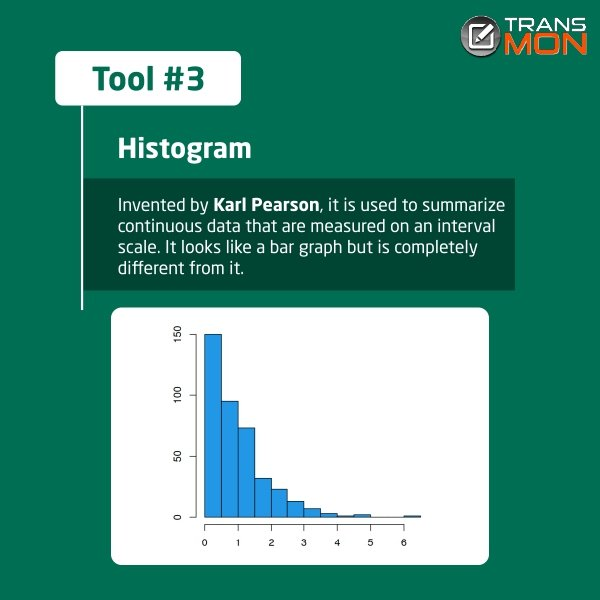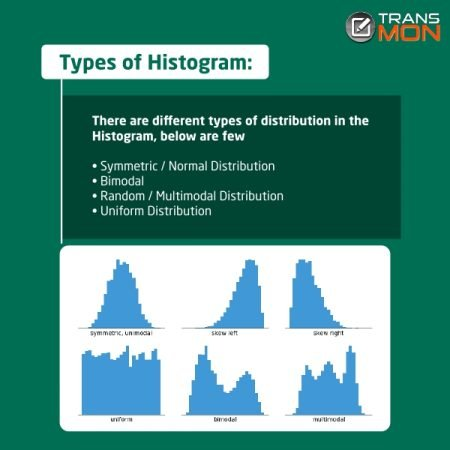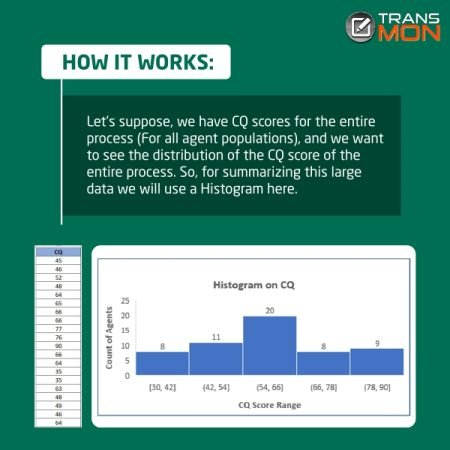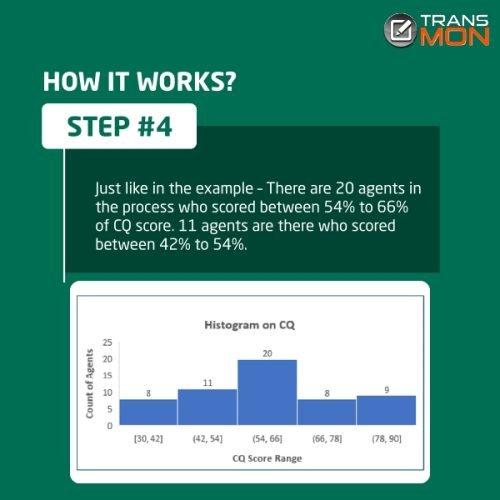The histogram is one of the 7 QC tools, which looks like a bar graph but is completely different from a bar graph (A bar graph is a one-dimensional graph whereas a Histogram is a two-dimensional one; the Visual feel is also a bit different as in Histogram there is no gap between the bars).

Invented by Karl Pearson, an English mathematician; The histogram is used to summarize continuous data that are measured on an interval scale.

• It represents the frequency distribution of specific data in the process
• It classifies data into various “bins” or “range groups” and counts how many data points belong to each of those bins.
• The X-axis (Horizontal)demonstrates grouped intervals (and not the individual points).
• The height of the bars (Y-axis/Vertical Axis) signifies the distribution frequency of a variable (the count, or how often that variable appears between that specific range). It shows you the frequency of the values within an interval.
• The width represents the length of the interval covered by the bar.### The histogram is useful

• For summarizing large data sets graphically.
• To evaluate process capability& understand variation.
• To distinguish whether a process is stable and predictable.
• Useful in determining the minimum data point, maximum data point, and median.

### There are different types of distribution in the Histogram, below are few

• Symmetric / Normal Distribution
• Bimodal
• Random / Multimodal Distribution
• Uniform Distribution### Example of Histogram

Let’s suppose, we have CQ scores for the entire process (For all agent populations), and we want to see the distribution of the CQ score of the entire process. So, for summarizing this large data we will use a Histogram here.

### How It Works

Step 1

• Select the data range and click on Insert Insert Statistic Chart - HistogramStep 2

• You can change the number of bins and range after clicking on horizontal axis- Format Axis - Number of Bins/Bin width as per your requirement.

Step 3

• From Histogram here you can identify the count of agents falling between any specific CQ range (shown on the graph).Step 4

• Just like in the above example – There are 20 agents in the process who scored between 54% to 66% of CQ score. 11 agents are there who scored between 42% to 54%.

### Eventual Outcome

A Histogram will group your data into Bins or Ranges, and then you can check your process health by looking at these bins – You can identify where your maximum population is falling, what is the contribution of the population who are exceeding the target, meeting the target, or not able to meet the target and set your action plan accordingly.